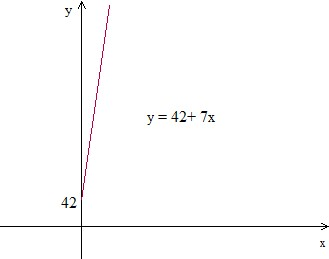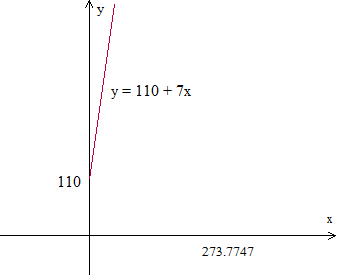# Writing an equation and drawing its graph to model a real-world situation: Basic

#### Excel Pivot Tables and Dashboard. Simple & Quick tutorial!

Most Popular

7 Lectures 58 mins

#### Learn HTML Tables

15 Lectures 59 mins

#### Excel Analytics - Data Analysis with Pivot-Tables and Charts

46 Lectures 2.5 hours

In this lesson, we solve real world problems which are modeled by an equation. The equation describing the problem is written and its graph is plotted.

Jenny has savings of $42 and earns$7 for each hour of lawn mowing. If y is the total amount with Jenny and x is the number of hours he works, write and equation in y and x and graph it.

### Solution

Step 1:

The equation representing the problem in x and y is

y = 42 + 7x

Step 2:

The plot isPhil's party costs $110 plus$7 for every guest he invites. Let y be the total cost of party and x be the number of guests. Write an equation in x and y and graph it.

### Solution

Step 1:

The equation representing the problem in x and y is y =110 + 7x

Step 2:

The plot is